Math Olympiad Test: Linear Educations in Two Variables- 1

# Math Olympiad Test: Linear Educations in Two Variables- 1

Test Description

## 10 Questions MCQ Test Mathematics Olympiad for Class 9 | Math Olympiad Test: Linear Educations in Two Variables- 1

Math Olympiad Test: Linear Educations in Two Variables- 1 for Class 9 2022 is part of Mathematics Olympiad for Class 9 preparation. The Math Olympiad Test: Linear Educations in Two Variables- 1 questions and answers have been prepared according to the Class 9 exam syllabus.The Math Olympiad Test: Linear Educations in Two Variables- 1 MCQs are made for Class 9 2022 Exam. Find important definitions, questions, notes, meanings, examples, exercises, MCQs and online tests for Math Olympiad Test: Linear Educations in Two Variables- 1 below.
Solutions of Math Olympiad Test: Linear Educations in Two Variables- 1 questions in English are available as part of our Mathematics Olympiad for Class 9 for Class 9 & Math Olympiad Test: Linear Educations in Two Variables- 1 solutions in Hindi for Mathematics Olympiad for Class 9 course. Download more important topics, notes, lectures and mock test series for Class 9 Exam by signing up for free. Attempt Math Olympiad Test: Linear Educations in Two Variables- 1 | 10 questions in 10 minutes | Mock test for Class 9 preparation | Free important questions MCQ to study Mathematics Olympiad for Class 9 for Class 9 Exam | Download free PDF with solutions
 1 Crore+ students have signed up on EduRev. Have you?
Math Olympiad Test: Linear Educations in Two Variables- 1 - Question 1

### The cost of a chair is half of the cost of a dining table. The linear equation representation of the above will be:

Detailed Solution for Math Olympiad Test: Linear Educations in Two Variables- 1 - Question 1

Let the cost of a chair be Rs. y and cost of dining table be Rs. x
According to the question
y = x/2
⇒ x = 2y

Math Olympiad Test: Linear Educations in Two Variables- 1 - Question 2

### The value of k, if, (3, 2) is a solution of equation 4x + y = k is:

Detailed Solution for Math Olympiad Test: Linear Educations in Two Variables- 1 - Question 2

Given 4x + y = k
∴ (3, 2) is a solution of above equation
∴ (3, 2) will satisfy the above equation
∴  4 x 3 + 2 = 12 + 2 = 14 = k

Math Olympiad Test: Linear Educations in Two Variables- 1 - Question 3

### If α2x + ay = 3, is satisfied by x = 1, y = 2, then the value of α will be:

Detailed Solution for Math Olympiad Test: Linear Educations in Two Variables- 1 - Question 3

Here (1, 2) α2x + αy - 3 = 0
then α2 (1) + α(2) - 3 = 0
⇒ α2 + 2α - 3 = 0
⇒ α2 + 3α - α - 3 = 0
⇒ α(α + 3) - 1(α + 3) = 0
⇒ (α + 3) (α - 1) = 0
⇒ α + 3 = 0, or α - 1 = 0
α = -3, or α = 1

Math Olympiad Test: Linear Educations in Two Variables- 1 - Question 4

The equation x - y + 1 = 0 is satisfied by x = α2 and y = α, then α =

Detailed Solution for Math Olympiad Test: Linear Educations in Two Variables- 1 - Question 4

2, a) is a solution of the equation
x - y + 1 = 0
⇒ α2 - a + 1 = 0
⇒ The above equation has negative  discriminant.
∴ value of a cannot be determined

Math Olympiad Test: Linear Educations in Two Variables- 1 - Question 5

If the equation x + 3y + 4k = 6 is satisfied by (2, 3) then the value of k is:

Detailed Solution for Math Olympiad Test: Linear Educations in Two Variables- 1 - Question 5

(2, 3) satisfies the equation x + 3y + 4k = 6,
then,
2 + 3 (3) + 4k = 6
⇒ 2 + 9 + 4k = 6
⇒ 4k = -5
⇒ k = -5/4

Math Olympiad Test: Linear Educations in Two Variables- 1 - Question 6

If the equation k (x3 - y3) = (x2 + y2 + xy) and y = 1k , then the value of x is

Detailed Solution for Math Olympiad Test: Linear Educations in Two Variables- 1 - Question 6

k (x3 - y3) = x2 + y2 + xy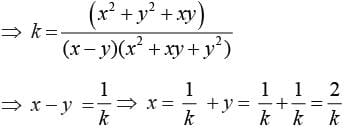Math Olympiad Test: Linear Educations in Two Variables- 1 - Question 7

The equation, (x + y) +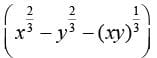= 12, then, u = ??, if x = 8

Detailed Solution for Math Olympiad Test: Linear Educations in Two Variables- 1 - Question 7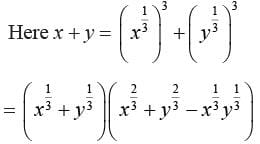⇒ The equatios will be reduced to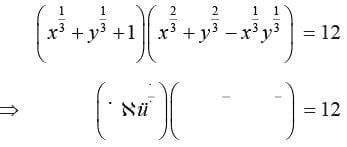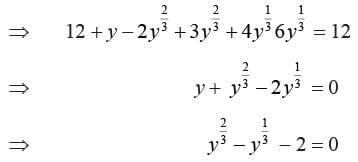Let ,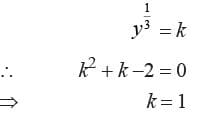Math Olympiad Test: Linear Educations in Two Variables- 1 - Question 8

Arun and Kajol together contributed 100 rupees for the Prime Minister Relief fund. If the money donated by Arun is Rs 80 less than twice the money donated by Kajol then the money donated by Arun is:

Detailed Solution for Math Olympiad Test: Linear Educations in Two Variables- 1 - Question 8

Let the amount donated by Kajol be rs x
∴ Amount donated by Arun = Rs. (2x - 80)
According to the question
x + 2x - 80 = 100
⇒ 3x = 180
⇒ x = 60
∴ Money donated by Arun = Rs (2 × 60 - 80) = Rs. 40

Math Olympiad Test: Linear Educations in Two Variables- 1 - Question 9

If the point (4, 5) lies on the graph 3y = ax + 3, then a =

Detailed Solution for Math Olympiad Test: Linear Educations in Two Variables- 1 - Question 9

Point (4, 5) lies on the graph of the equation 3y = αx + 3
∴  3 × 5 = 4α + 3
⇒ 4α = 12
⇒ α = 3

Math Olympiad Test: Linear Educations in Two Variables- 1 - Question 10

If C =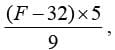where C denotes the temperature in Celsius and F denotes the temperature in Fahrenheit, The temperature (in Celsius) at which the numerical value on the both scales is same will be

Detailed Solution for Math Olympiad Test: Linear Educations in Two Variables- 1 - Question 10

Let the numerical value of temperature be x
∴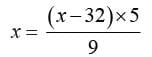⇒ 9x = 5x -160
⇒ 4x = -160
⇒ x = -40
∴ the temperature is equal in both the scales at -40°C.

## Mathematics Olympiad for Class 9

1 videos|43 tests
 Use Code STAYHOME200 and get INR 200 additional OFF Use Coupon Code
Information about Math Olympiad Test: Linear Educations in Two Variables- 1 Page
In this test you can find the Exam questions for Math Olympiad Test: Linear Educations in Two Variables- 1 solved & explained in the simplest way possible. Besides giving Questions and answers for Math Olympiad Test: Linear Educations in Two Variables- 1, EduRev gives you an ample number of Online tests for practice

## Mathematics Olympiad for Class 9

1 videos|43 tests